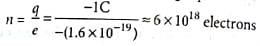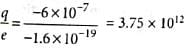# NCERT Based Test: Basic Properties of Electric Charge

## 5 Questions MCQ Test NCERTs for NEET | NCERT Based Test: Basic Properties of Electric Charge

Description
Attempt NCERT Based Test: Basic Properties of Electric Charge | 5 questions in 5 minutes | Mock test for NEET preparation | Free important questions MCQ to study NCERTs for NEET for NEET Exam | Download free PDF with solutions
QUESTION: 1

### The number of electrons present in -1C of charge is

Solution:

By quantisation of charge, q = ne
orQUESTION: 2

### A cup contains 250 g of water. Find the total positive charges present in the cup of water.

Solution:

Mass of water = 250 g,
Molecular mass of water = 18 g
Number of molecules in 18 g of water = 6.02 x 1023
Number of molecules in one cup of water = (250/18) x 6.02 x 1023
Each molecule of water contains two hydrogen atoms and one oxygen atom, i.e., 10 electrons and 10 protons.
∴ Total positive charge present in one cup of water
= (250/18) x 6.02 x 1023 x 10 x 1.6 x 10-19 C = 1.34 x 107C.

QUESTION: 3

### A polythene piece rubbed with wool is found to have a negative charge of 6 x 10-7 C. The number of electrons transferred to polythene from wool is

Solution:

Here, q = -6 x 10-7 C
∴ Number of electrons transferred from wool to polythene, n =QUESTION: 4

If 109 electrons move out of a body to another body every second, then the time required to get charge of 1 C on the other body is

Solution:

The charge given out in one second = 1.6 x 10-19 C x 109 = 1.6 x 10-10C
Time required to accumulate a charge of 1 C
= 1/(1.6 x 10-10) = 6.25 x 109 s = 198 years

QUESTION: 5

The number of electrons that must be removed from an electrically neutral silver dollar to give it a charge of +2.4 C is

Solution:

Total charge, q = 2.4 C
Then by quantization of charge, q = ne
∴ number of electrons, n = q/e = 2.4C/1.6 x 10-19 C = 1.5 x 1019Use Code STAYHOME200 and get INR 200 additional OFF Use Coupon Code